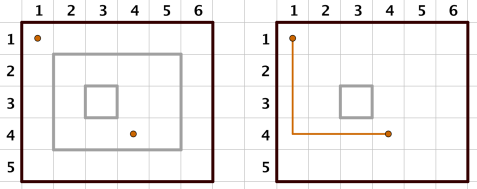# CF869E The Untended Antiquity 题解【树状数组】【哈希】

二维树状数组的模板题……之前写的时候用二维线段树水过了OI版的，CF数据要强很多。

## Description

Koyomi is helping Oshino, an acquaintance of his, to take care of an open space around the abandoned Eikou Cram School building, Oshino’s makeshift residence.

The space is represented by a rectangular grid of $n\times m$ cells, arranged into $n$  rows and $m$ columns. The $c$-th cell in the $r$-th row is denoted by $(r,c)$.

Oshino places and removes barriers around rectangular areas of cells. Specifically, an action denoted by “$1\ r_1\ c_1\ r_2\ c_2$” means Oshino’s placing barriers around a rectangle with two corners being $(r_1,c_1)$ and $(r_2, c_2)$ and sides parallel to squares sides. Similarly, “$2\ r_1\ c_1\ r_2\ c_2$” means Oshino’s removing barriers around the rectangle. Oshino ensures that no barriers staying on the ground share any common points, nor do they intersect with boundaries of the $n\times m$ area.

Sometimes Koyomi tries to walk from one cell to another carefully without striding over barriers, in order to avoid damaging various items on the ground. “$3\ r_1\ c_1\ r_2\ c_2$” means that Koyomi tries to walk from $(r_1, c_1)$ to $(r_2, c_2)$ without crossing barriers.

And you’re here to tell Koyomi the feasibility of each of his attempts.

## Input

The first line of input contains three space-separated integers $n, m$ and $q (1 ≤ n, m ≤ 2 500, 1 ≤ q ≤ 100 000)$ — the number of rows and columns in the grid, and the total number of Oshino and Koyomi’s actions, respectively.

The following $q$ lines each describes an action, containing five space-separated integers $t, r_1, c_1, r_2, c_2 (1 ≤ t ≤ 3, 1 ≤ r_1, r_2 ≤ n, 1 ≤ c_1, c_2 ≤ m)$ — the type and two coordinates of an action. Additionally, the following holds depending on the value of $t$:

• If $t = 1: 2 ≤ r_1 ≤ r_2 ≤ n - 1, 2 ≤ c_1 ≤ c_2 ≤ m - 1$;
• If $t = 2: 2 ≤ r_1 ≤ r_2 ≤ n - 1, 2 ≤ c_1 ≤ c_2 ≤ m - 1$, the specified group of barriers exist on the ground before the removal.
• If $t = 3$: no extra restrictions.

## Output

For each of Koyomi’s attempts (actions with $t = 3$), output one line — containing “Yes” (without quotes) if it’s feasible, and “No” (without quotes) otherwise.

## Examples

input
5 6 5
1 2 2 4 5
1 3 3 3 3
3 4 4 1 1
2 2 2 4 5
3 1 1 4 4
output
No
Yes
input
2500 2500 8
1 549 1279 1263 2189
1 303 795 1888 2432
1 2227 622 2418 1161
3 771 2492 1335 1433
1 2017 2100 2408 2160
3 48 60 798 729
1 347 708 1868 792
3 1940 2080 377 1546
output
No
Yes
No

## Note

For the first example, the situations of Koyomi’s actions are illustrated below.## 题意：

在一个长$n$宽$m$的大矩形中，有三种操作，第一种：砌一堵墙；第二种：把之前建过的墙拆掉一个；第三种：询问两个点能否相互到达。保证墙与墙、边界互不相交。

## 题解：

当时在ASDFZ考过这个题，因为数据很难造，所以各种乱搞可以过。这里如果两个格子可以互达，那么覆盖它们的矩形种类、个数一定完全相同。此时我们可以用一个$base$来区分每个矩形，比如第一个矩形是$base^1$，第二个是$base^2$，……这样再取一个特殊的模数或者自动溢出，就有了每个联通块独一无二的特征值。特征值是可以通过二维树状数组来加减的。

二维树状数组的操作很简单，只需要横坐标跑一遍，并嵌套纵坐标跑一遍就可以了，树状数组中也要注意取模。不过要注意，这里是相当于二维，因此差分的减标记也是要打一排的。

去掉墙的操作可以直接存一下以这个点为左上角的矩形的编号，那么给这个矩形也减去$base^{编号}$就可以了。

## Code：

#include<cstdio>
#include<cstring>
long long p=1000000007;
long long c;
int lowbit(int x)
{
return x&(-x);
}
int n,m;
void change(int x,int y,long long w)//二维修改
{
while(x<=n)
{
int Y=y;
while(Y<=m)
{
c[x][Y]=((c[x][Y]+w)%p+p)%p;
Y+=lowbit(Y);
}
x+=lowbit(x);
}
}
{
long long ans=0;
while(x)
{
int Y=y;
while(Y)
{
ans=(ans+c[x][Y])%p;
Y-=lowbit(Y);
}
x-=lowbit(x);
}
return ans;
}
long long qpow(int x)//方便取特征值
{
long long ans=1,m=3;
while(x)
{
if(x&1)
ans=ans*m%p;
m=m*m%p;
x>>=1;
}
return ans;
}
long long bs;
int main()
{
scanf("%d%d",&n,&m);
int q,op,lx,ly,rx,ry;
scanf("%d",&q);
while(q--)
{
scanf("%d%d%d%d%d",&op,&lx,&ly,&rx,&ry);
if(op==1)
{
long long base=qpow(q);
change(lx,ly,base);
change(lx,ry+1,-base);
change(rx+1,ly,-base);
change(rx+1,ry+1,base);
bs[lx][ly]=base;
}
else if(op==2)
{
long long base=bs[lx][ly];
change(lx,ly,-base);
change(lx,ry+1,base);
change(rx+1,ly,base);
change(rx+1,ry+1,-base);
}
else
{
puts("Yes");
else
puts("No");
}
}
return 0;
}

### 说点什么Subscribe

/* */